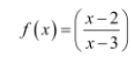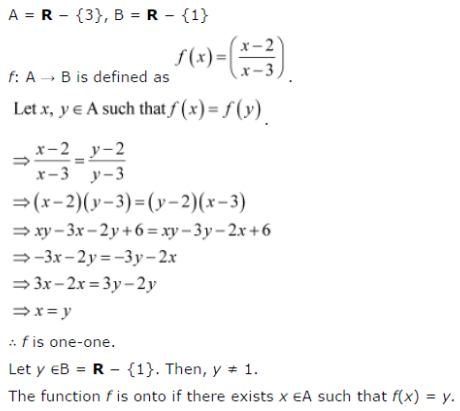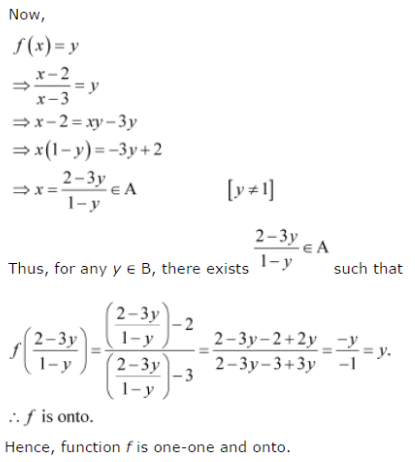# Let A = R – {3} and B = R – {1}. Consider the function f : A → B defined by

Let A = R – {3} and B = R – {1}. Consider the function f : A → B defined by